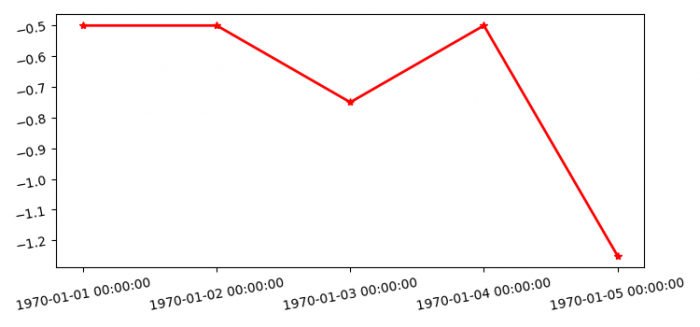# How to set the xticklabels for date in matplotlib?

To set the xticklabels for date in matplotlib, we can take the following steps −

## Steps

• Set the figure size and adjust the padding between and around the subplots.

• Create two lists of epochs and values.

• Get a list of dates from epochs.

• Create a figure and a set of subplots.

• Plot the date and values using plot() method.

• Set the xticklabels, get date formatter and set the major formatter.

• To remove the overlapping for ticklabels, rotate it by 10.

• To display the figure, use show() method.

## Example

import matplotlib.pyplot as plt
import matplotlib.dates as mdates
import time

plt.rcParams["figure.figsize"] = [7.50, 3.50]
plt.rcParams["figure.autolayout"] = True

epochs = [1259969793926, 1259969793927, 1259969793929, 1259969793928, 1259969793939]
values = [-0.5, -0.5, -0.75, -0.5, -1.25]

dates = [time.strftime('%Y-%m-%d %H:%M:%S', time.localtime(date)) for date in epochs]

fig, axes = plt.subplots(1, 1)
line1, = axes.plot(dates, values, lw=2, marker='*', color='r')

axes.set_xticklabels(dates)
fmt = mdates.DateFormatter('%Y-%m-%d %H:%M:%S')

axes.xaxis.set_major_formatter(fmt)
axes.tick_params(rotation=10)

plt.show()

## Output

It will produce the following output −